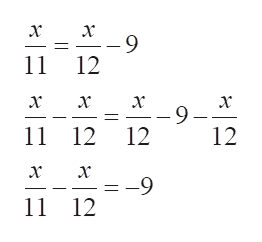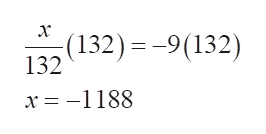How do you solve this equation?x/11=x/12-9

Question

How do you solve this equation?

x/11=x/12-9

Step 1

To solve an equation all the variables are to be brought to one side of the equation:

Subtracting x/12 on both the sides:help_outlineImage Transcriptionclosex -9 11 12 x x x x -9 12 12 11 12 x --9 11 12 fullscreen
Step 2

Here the variable terms have rational coefficients. To simplify the equation, the denominators of the rational terms need to be equal. So the LCD of the denominators is to be calculated and simplified:

Step 3

To isolate the variable x, 132 is mult...help_outlineImage Transcriptionclosex (132) = -9(132) 132 x -1188 fullscreen

Want to see the full answer?

See Solution

Want to see this answer and more?

Our solutions are written by experts, many with advanced degrees, and available 24/7

See Solution
Tagged in

Equations and In-equations